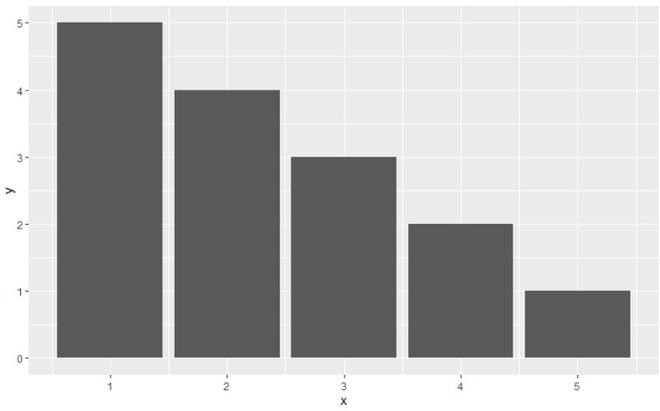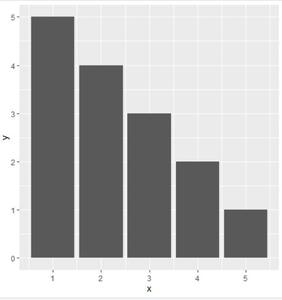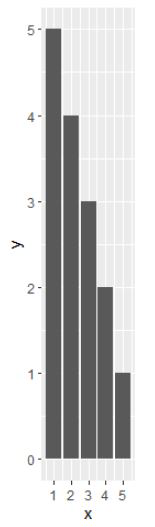Related Articles

# How to fix aspect ratio in ggplot2 Plot in R ?

• Last Updated : 17 Jun, 2021

In this article, we will be looking at the approach to fix the aspect ratio in the ggplot2 plot using functions in the R programming language.

The aspect ratio of a data graph is defined as the height-to-width ratio of the graph’s size. It can be fixed automatically using the coord_fixed() function when called with appropriate data.

A fixed scale coordinate system forces a specified ratio between the physical representation of data units on the axes. The ratio represents the number of units on the y-axis equivalent to one unit on the x-axis. The default, ratio = 1, this function is used to fix the aspect ratio.

Syntax:

coord_fixed(ratio = 1, xlim = NULL, ylim = NULL, expand = TRUE, clip = “on”)

Parameters:

• ratio: aspect ratio, expressed as y / x
• xlim: Limits for the x and y axes.
• ylim: Limits for the x and y axes.
• expand: If TRUE, the default, adds a small expansion factor to the limits to ensure that data and axes don’t overlap.
• clip: Should the drawing be clipped to the extent of the plot panel?

Let us see what the plot will appear without the aspect ratio set so that the difference is apparent.

Example:

## R

 `library``(``"ggplot2"``)   `` ` `gfg_data<-``data.frame``(x=``c``(1,2,3,4,5),y=``c``(5,4,3,2,1))`` ` `gfg_plot<-``ggplot``(data=gfg_data, ``aes``(x, y)) +``  ``geom_bar``(stat=``"identity"``)`` ` `gfg_plot`

Output:Now to fix the aspect ratio simply call it with the plot.

Example 1:

## R

 `library``(``"ggplot2"``)   `` ` `gfg_data<-``data.frame``(x=``c``(1,2,3,4,5),y=``c``(5,4,3,2,1))`` ` `gfg_plot<-``ggplot``(data=gfg_data, ``aes``(x, y)) +``  ``geom_bar``(stat=``"identity"``)``gfg_plot + ``coord_fixed``()`

Output:It is also possible to fix it explicitly. Just passing the argument ratio to the corrd_fixed() function to fix the ggplot2 plot as per the user requirements will do the job.

Example 2:

## R

 `library``(``"ggplot2"``)   `` ` `gfg_data<-``data.frame``(x=``c``(1,2,3,4,5),y=``c``(5,4,3,2,1))`` ` `gfg_plot<-``ggplot``(data=gfg_data, ``aes``(x, y)) +``  ``geom_bar``(stat=``"identity"``)`` ` `gfg_plot + ``coord_fixed``(5)`

Output:My Personal Notes arrow_drop_up